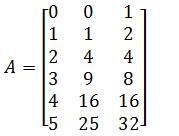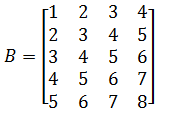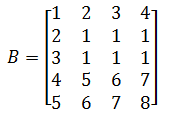Home MathLab Matlab Tricks: Creating an “Array”, Modifying Matrix Elements

# Matlab Tricks: Creating an “Array”, Modifying Matrix Elements

Among other tricks you can make use of while using Matlab, here are two tricks that may help you have easier control while working with Matrices.

The first tip helps you create a list of elements in a certain order, and the second will simply help you modify an element in an already existing matrix.

Here we go!

## Creating an Array/table of elements

Just to have more light toward the direction we are heading to with this tip, we will use the following example to show you what we are trying to build

We want to create something like the following

### Example 1### Example 2With these matrices, we are just trying to show that there is a certain pattern here which is somehow obvious from the second example. Elements of the matrix are equally spaced from a row/column to the next, for instance on the second example, the first column is 1 to 5 spaced from one element to the other with 1.

Let’s now look at how you can create such Matrices in Matlab

#### The code

Example 1 (code corresponding to example 1)

```n=(0:5)';
A=[n n.^2 2.^n]```

Example 2 (code corresponding to example 2)

```n=(1:5)';
B=[n n+1 n+2 n+3]```

In the examples above, you can change the steps size to less than one, by putting its value in between the digits in the first line of code

```n=(1:0.5:5) % the step size is now reduced to half
%The Matrix will have more rows if you do use this```

## Modifying Matrix elements

From previous posts on Matrix manipulation in Matlab, we know how to access specific elements in a Matrix, we can use the same technique to change the values of elements in a matrix.

If we have a Matrix A looking like the followingWe can, for instance, decide to change the second and third rows and the second, third and fourth column to 1.

#### The code

`B(2:3,2:4)=1`

Here is what B will look like then